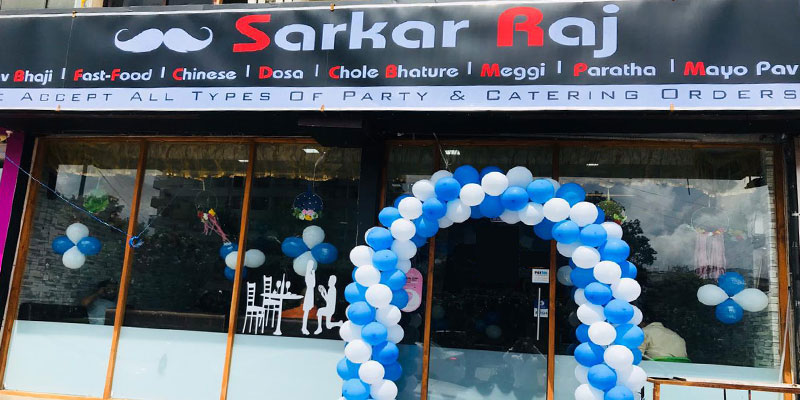# Sarkar Raj

#### Location: Piplod

South Indian     Chinese     Pizza     Fast Food

### TIMING · Open Now

(Wed) 11:00 AM to 09:30 PM

S3-S4, Vaishnodevi Complex, Kargil Chowk, Piplod, Surat

Find on Map

## Offers and Discount in Sarkar Raj

Get 1 Veg Cheese Grill Sandwich (Rs. 120) + 1 Veg Sandwich (Rs. 50) = Rs. 170 At Rs. 150

Get 1 Mysore Dosa (Rs. 120) + 3 Plain Paper Dosa (Rs. 50 X 3 = 150) = Rs. 270 At Rs. 150

Get 1 Dry Manchurian (Rs. 120) + 1 Fried Rice (Rs. 100) = Rs. 220 At Rs. 150

Get 1 Pavbhaji (Rs. 120) + 1 Tawa Pulav (Rs. 120) = Rs. 240 At Rs. 180

Get 1 Special Chinese Bhel (Rs. 180) + 1 Cheese Mayo Pav (Rs. 40) = Rs. 220 At Rs. 200

Get 1 Sp. Sarkar Pizza (Rs. 180) + 1 Garlic Bread (Rs. 100) = Rs. 280 At Rs. 200

Get 2 Masala Dosa (Rs. 100 X 2 = 200) + 1 Paper Dosa (Rs. 50) = Rs. 250 At Rs. 200

Get 1 Jini Roll Dosa (Rs. 180) + 1 Cheese Paper Dosa (Rs. 110) = Rs. 290 At Rs. 200

Get 1 Paneer Chilli (Rs. 150) + 1 Hakka Noodles (Rs. 100) = Rs. 250 At Rs. 200

Get 1 Triple Schezwan Rice (Rs. 150) + 1 Dry Manchurian (Rs. 120) = Rs. 270 At Rs. 220

Buy Any 1 Ice Dish (Rs. 100) & Get 1 Ice Gola (Rs. 50 - 60) Free